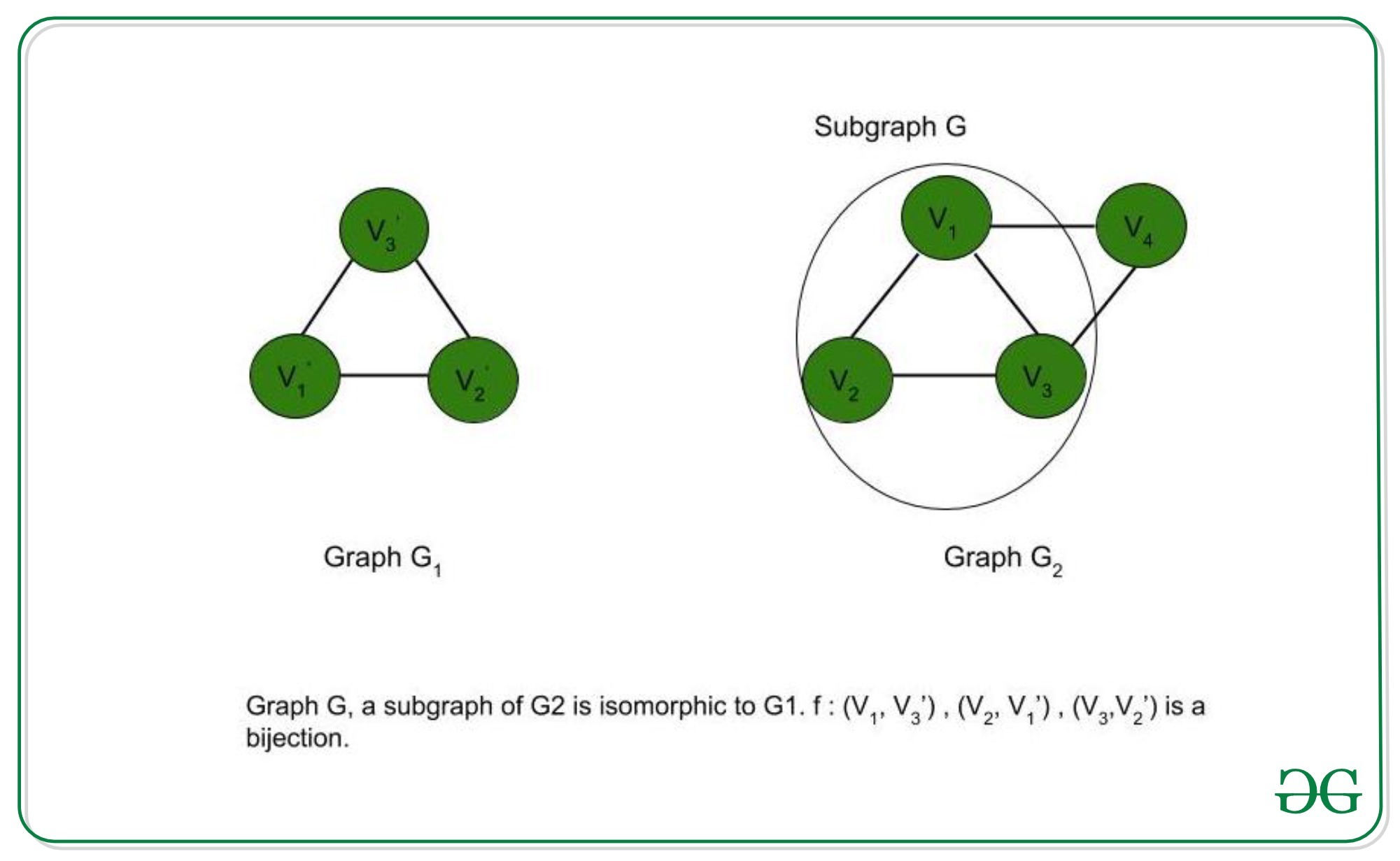GeeksforGeeks App
Open AppBrowser
Continue

# Proof that Subgraph Isomorphism problem is NP-Complete

Subgraph Isomorphism Problem: We have two undirected graphs G1 and G2. The problem is to check whether G1 is isomorphic to a subgraph of G2.

Graph Isomorphism: Two graphs A and B are isomorphic to each other if they have the same number of vertices and edges, and the edge connectivity is retained. There is a bijection between the vertex sets of the graphs A and B. Hence, two vertices u, v are adjacent to each other in A if and only if f(u), f(v) are adjacent in B (f is a bijection).

To prove that a problem is NP-Complete, we have to show that it belongs to both NP and NP-Hard Classes. (Since NP-Complete problems are NP-Hard problems which also belong to NP)The Subgraph Isomorphism Problem belongs to NP – If a problem belongs to the NP class, then it should have polynomial-time verifiability. Given a certificate, we should be able to verify in polynomial time if it is a solution to the problem.

Proof:

1. Certificate: Let G be a subgraph of G2. We also know the mapping between the vertices of G1 and G.
2. Verification: We have to check if G1 is isomorphic to G or not. (i) Checking if the mapping is a bijection and (ii) Verifying if, for every edge (u, v) in G1, there is an edge (f(u), f(v)) present in G takes polynomial time.

Therefore, the Subgraph Isomorphism Problem has polynomial time verifiability and belongs to the NP class.

The Subgraph Isomorphism Problem belongs to NP-Hard –A problem L belongs to NP-Hard if every NP problem is reducible to L in polynomial time. To prove that the Subgraph Isomorphism Problem (S) is NP-Hard, we try to reduce an already known NP-Hard problem to S for a particular instance. If this reduction is possible in polynomial time, then S is also an NP-Hard problem. Thus, let us reduce the Clique Decision Problem (C) which is NP-Complete (hence, all the problems in NP can be reduced to C in polynomial time) to the Subgraph Isomorphism Problem. If this reduction is possible in polynomial time, every NP problem can be reduced to S in polynomial time, thereby proving S to be NP-Hard.

Proof: Let us prove that the Clique Decision Problem reduces to the Subgraph Isomorphism Problem in polynomial time.

Let the input to the Clique Decision Problem be (G, k). The output is true if the graph G contains a clique of size k (a clique of size k is a subgraph of G). Let G1 be a complete graph of k vertices and G2 be G, where G1, G2 are inputs to the Subgraph Isomorphism Problem. We observe that k <=n, where n is the number of vertices in G (=G2). If k>n, then a clique of size k cannot be a subgraph of G. The time taken to create G1 is O(k2) = O(n2) [since k<=n] as the number of edges in a complete graph of size k = kC2 = k*(k-1)/2. G has a clique of size k, if and only if G1 is a subgraph of G2 (since G1 itself is a subgraph of G2 and every graph is isomorphic to itself, the result of the Subgraph Isomorphism Problem is true. Thus, G1 is isomorphic to a subgraph of G2). Hence, if the Clique Decision Problem is true, the Subgraph Isomorphism Problem is true and vice versa. Therefore, the Clique Decision Problem can be reduced to the Subgraph Isomorphism Problem in polynomial time for a particular instance.

Thus, the Subgraph Isomorphism Problem is an NP-Hard Problem

Conclusion:
The Subgraph Isomorphism Problem is NP and NP-Hard. Therefore, the subgraph isomorphism problem is NP-Complete.

My Personal Notes arrow_drop_up